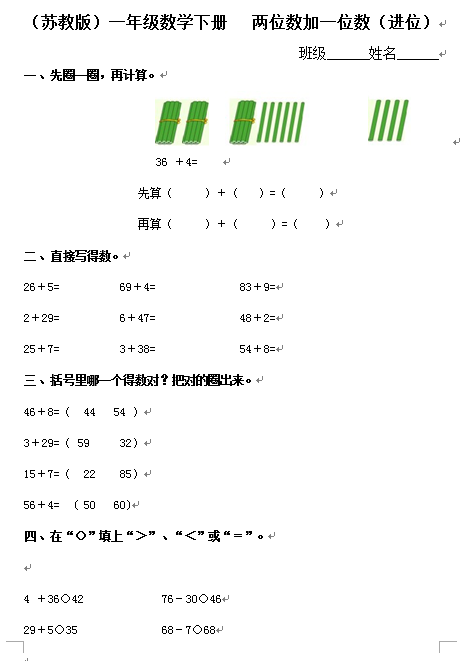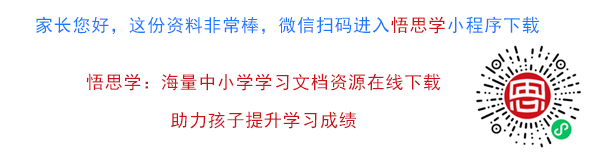36 ＋4=

26＋5=          69＋4=              83＋9=

2＋29=          6＋47=              48＋2=

25＋7=          3＋38=              54＋8=

【DOC文档4页】（苏教版）一年级数学下册 两位数加一位数（进位）及答案 A4电子版资料_可直接打印_会员免费下载##### 评论信息